Journal of Electrical and Electronic Engineering
Volume 4, Issue 2, April 2016, Pages: 40-43

Towards a Precise Direction of Arrival Estimation for Coherent Sources Using EC-MUSIC

Hassan Mohamed EL-Kamchouchi, Ahmed Samir EL-Torgoman

Electrical Engineering, Alexandria University, Alexandria, Egypt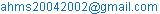(H. M. EL-Kamchouchi)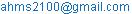(A. S. EL-Torgoman)

Hassan Mohamed EL-Kamchouchi, Ahmed Samir EL-Torgoman. Towards a Precise Direction of Arrival Estimation for Coherent Sources Using EC-MUSIC. Journal of Electrical and Electronic Engineering. Vol. 4, No. 2, 2016, pp. 40-43. doi: 10.11648/j.jeee.20160402.17

Received: March 25, 2016; Accepted: April 6, 2016; Published: April 19, 2016

Abstract: Smart antennas are used to transmit /receive the signals according smart choices due to DSP, the most important factor in this choices is signal direction for reception or transmission, known as DoA: direction of arrival, there are much many algorithms used to address this issue as MUSIC/ROOT MUSIC/ESPIRIT /MVDR In this paper we will concentrate on MUSIC algorithm are one of the most attractive algorithm regarding its performance, However 2 or more signals are close to each other these algorithm do not perform accurate, as well as under coherent sources the accuracy deteriorates quickly in this paper a reasonable modification for MUSIC had been made by adding coefficients related to number of snapshots and number of element array  as well as simple modification for covariance matrix in MUSIC algorithm to capable to handle signals of coherent sources will result in enhancing MUSIC algorithm resolution for coherent sources to 1 degree space which will be called EC-MUSIC (Enhanced Coherent-MUSIC).

Keywords: Smart Antennas, Direction of Arrival, Coherent Sources

Contents

1. Introduction

Smart antennas combine multiple antenna elements with signal processing capability to optimize radiation / reception  and is divided to two main sections beam forming signal is formed due to signal coming from target which decrease power consumption and nulls interferers jamming signal and frequency reuse will be used within cell space called space division multiple access SDMA , the second section of smart antenna is direction of arrival which is so important for finding the direction from which the signal is coming from many algorithms are studied in this area [1,4,5,6], it is important in many applications like sonar/rescue devices wireless communication, algorithms addressing this technique divided into three main categories conventional & beam forming and subspace techniques as  MUSIC/ESPIRIT/BARTLETT/CAPON, the implementation is used to eliminate interference & combine the required signals to improve performance, from literature the common problems for this algorithm 2 adjacent signals cannot be detected however MUSIC is more better than Bartlett, MVDR and linear prediction method in this paper we will address the problem of coherent sources with MUSIC algorithm by inserting transition matrix to correct

covariance matrix de efficient a more simpler way than spatial smoothing method as well as enhancing resulting resolution by inserting enhancement Coefficients as no of snapshots, no of elements, which will result in enhancing MUSIC algorithm resolution for coherent sources to 1 degree space which will be called EC-MUSIC (Enhanced coherent-MUSIC) The paper is organized as follows Road map, Presentation of basic MUSIC algorithm. coherent sources and its problem of detection. how it is solved. presentation of enhancing Coefficients, seeing the results, conclusion and future work

We can simplify the roadmap in the following points.

A. Coherent MUSIC Overview

B. Basic MUSIC Algorithm

C. Coherent Sources problem in MUSIC

D Solving Methodology

E. Enhancing Resolution

F. Simulation steps and results

G. Conclusion

H. Future Work

3. Coherent MUSIC Overview

Coherent MUSIC based on MUSIC algorithm in which transition matrix is conjugate construction of the data matrix (Covariance matrix) of the MUSIC algorithm.

3.1. Basic MUSIC Algorithm

MUSIC: stands for Multiple SIgnal Classification it has been firstly presented by Schmidt  to estimate DOA it depends mainly on covariance Matrix of data from which Eigen vector for signal and noise using the data model in equation.

X = Sα + n               (1)

Where

S = [s((ϕ1), s(ϕ2), …, s(ϕM)]

α = [α1, α2, . . αM]T

The Matrix S is N * M where M is steering vector and N is the number of elements assuming signals are to be un correlated the correlation matrix of x is written as

R = E [XXH]                  (2)

= E[SααHSH]+E[nnH],         (3)

= SASH+ σ2I               (4)

= RS + σ2I                 (5)

Where

RS=SAS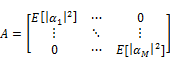The signal covariance, Rs. is clearly N×N with rank M. It therefore has N-M eigen vectors corresponding to the zero Eigen value let qm such eigen vector therefore

RSqm= SASHqm =0            (6)

qmH SASH qm =0

SHqm=0                       (7)

Where this final equation is valid since the matrix A is clearly positive definite. Equation implies that all N-M eigen vectors (qm) of RS corresponding to the zero eigenvalue are orthogonal to all M signal steering vectors.

This is the basis for MUSIC. Call Qn the N ×(N M) matrix of these eigenvectors. MUSIC plots the pseudo-spectrum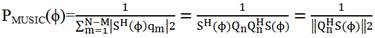(8)

Note that since the eigenvectors making up Qn are orthogonal to the signal steering vectors, the denominator becomes zero when ϕ is a signal direction.

3.2. Coherent Sources Overview

The problem involving coherent sources is a fatal problem for subspace algorithms . When there is a coherent signal in the signal source, the signal covariance matrix is rank defective. In this case, the original super-resolution algorithm will fail. Therefore, it will greatly affect the performance of DOA estimation.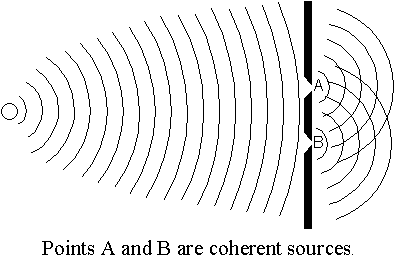Figure 1. Coherent Sources illustration .

3.3. Coherent Sources Problem in MUSIC

Highly correlated or coherent source signals are common in multipath propagation environments due to the reflection and refraction of source signals in practical. Based on such scenario, the coherent sources facilitate the rank loss of the covariance matrix, which could result in the failure of the conventional high-resolution estimation algorithms.

Covariance matrix rank loss is addressed by many methods to overcome its bad effect in Direction of arrival estimation. A simple method which was proposed by  conjugate reconstruction of covariance matrix of MUSIC algorithm.

3.3.2. Methodology

Make a transformation matrix J, J is an Mth-order anti-matrix, known as the transition matrix, i. e.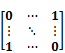Let Y=JX*, where X* is the complex conjugate of X, then the covariance of data matrix Y is

Ry=E [YYH] =JRX*J.                (9)

From the sum of Rx and Ry, the reconstructed conjugate matrix can be obtained.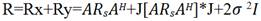(10)

According to matrix theory, the matrices Rx, Ry and R have the same noise subspace. To conduct characteristic decomposition of R and get its eigenvalue and eigenvector, according to the estimated number of signal source, separate the noise subspace, and then use this new noise subspace to construct spatial spectrum and obtain the estimated DOA value by finding the peak.

4. Enhancing Resolution

The last phase in this method to enhance resolution of very close angles of DoA When the separation angles between sources are very small, MUSIC algorithm couldn’t estimate the angles correctly. Thus an improvement for that algorithm was proposed by adding new coefficients to achieve this goal and estimate the adjacent angles correctly. This was achieved even with separation of degree only between sources. These coefficients are related to signal and antenna parameters which are affecting the accuracy of MUSIC algorithm. Those coefficients represent the ratio by which each corresponding parameter should be increased in order to get the required super high resolution. Those coefficients will virtually maximize the values of the corresponding parameters during calculation and enhance the estimation accuracy of the algorithms. Those coefficients are defined as follows as

4.1. Snapshots Coefficient (SC)

In order to increase the efficiency of estimation and obtain sharper peaks in MUSIC. A higher number of snapshots is required. Incrementing snapshots by snapshots Coefficient which is greater than one and multiplied by basic number of snapshots. The estimation variance for covariance matrix will decrease and thus sharper peaks for MUSIC are obtained. However, very high values for SC will lead to processing delay since the processing time will also increase. Hence, suitable value for it should be selected according to the requirements the new number of snapshot N′ can then be written as

N′ = N × SC                  (11)

4.2. Elements Coefficient (EC)

For more signal component in estimation result to clarify signal peaks for adjacent coherent signals. Element coefficient if multiplied by original elements number of array M. Thus, it gives more signal component for the algorithm. Here the importance of this modification appears during manufacturing process. By selecting the suitable EC value, the suitable number of elements required for the antenna design can be selected easily. Then we can substitute the new number of element M′ in MUSIC. M′ can be expressed by:

M= M × EC                  (12)

5. Simulation Steps & Results

Simulation done with MATLAB ®2015a release to clarify obtained results we have to see it compared with results obtained without any improvement with the raw MUSIC algorithm as a main frame. We will begin with incoherent sources and Raw MUSIC algorithm with 2 direction of arrival incidence (30°, 70°) degrees as in figure 2, for snapshots mainly =200 and number of element array (Uniform linear array)= 10.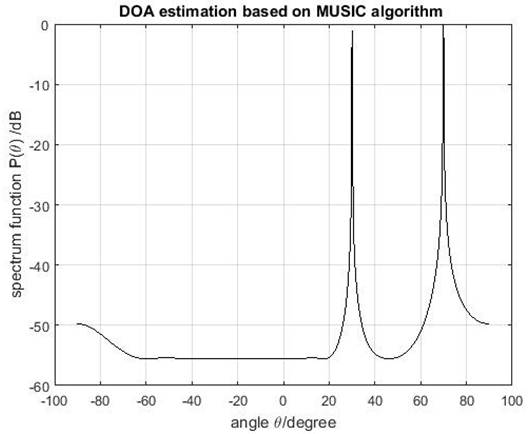Figure 2. Incoherent sources and raw MUSIC.

Then coherent sources with the same frequency and same incidence angles as last case and raw MUSIC algorithm we find performance degradation as in figure 3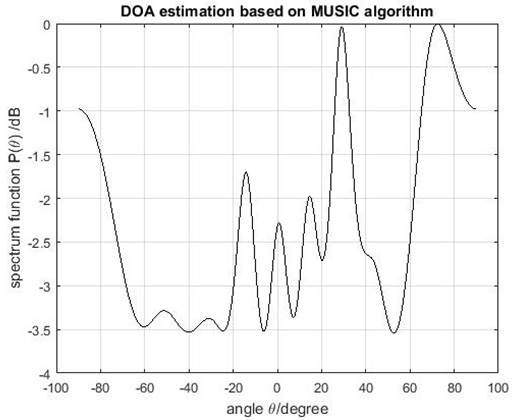Figure 3. Coherent raw with basic MUSIC.

Then check again with coherent MUSIC algorithm the peaks appear only at the estimated angles with high accuracy as seen in figure 4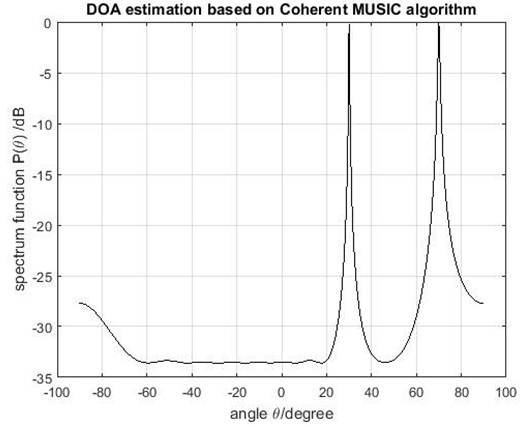Figure 4. Coherent sources with coherent MUSIC.

But when incident angles is adjacent (30°, 31°) the coherent MUSIC algorithm failed to discriminate them as in figure 5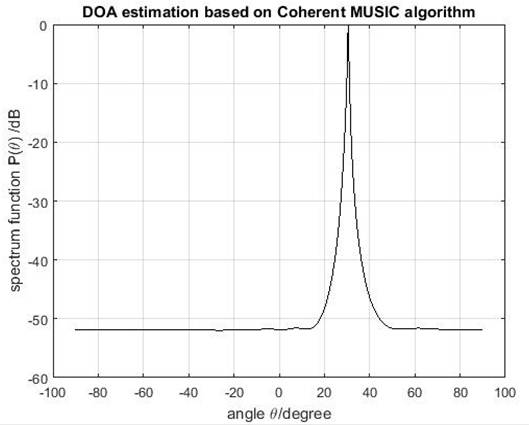Figure 5. Adjacent coherent sources with coherent MUSIC without enhancing resolution.

But when we use Enhanced coherent MUSIC with enhancing resolution Coefficients we find incredible accuracy as we see in the zoomed figure 6.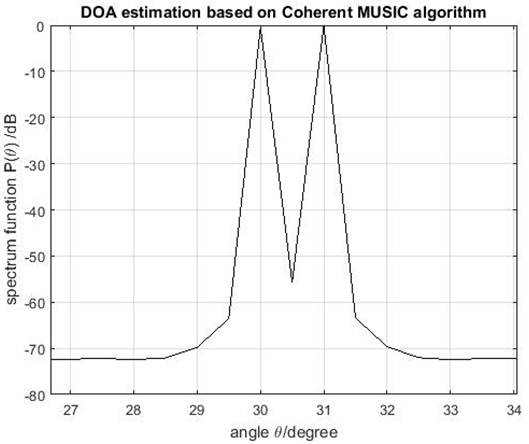Figure 6. Adjacent coherent signal estimation with EC-MUSIC (Zoom)with EC=3 ;and SC=1.5.

6. Conclusion

In this paper the theory of smart antennas and the basic concept of MUSIC DOA algorithms had been illustrated and discussed. That algorithm when implemented in smart antenna will lead to efficient use of networks. To achieve this goal, the estimation of source direction should be accurate even if sources are too close to each other's in angles and coherent which is achieved through covariance matrix correction and adding enhancing coefficients that led at last to accurate estimation for direction of arrival for adjacent coherent signals. However, excessive increment for coefficients values will lead to degradation in performance so that; suitable values for coefficients should be selected.

7. Future work

Enhancing resolution of DoA for coherent signals is now an important demand in today’s world of smart antennas, this work can be modified to update root MUSIC algorithm as well as Mixed signals identification in Multipath environment will be promising land for further research.

Acknowledgements

I would like to thank my Professor and Co-author Hassan. M EL-Kamchouhi For his un conditional scientific support.

References

1. Z. Chen, G. Gokeda, and Y. Q. Yu. Introduction to Direction-of-arrival Estimation. Artech House, (2010).
2. Y. Liao, A. Abouzaid. Resolution Improvement for MUSIC and ROOT MUSIC Algorithms(2015).
3. F. Gross. Smart antennas for wireless communications: with MATLAB, McGraw-Hill, (2005).
4. M. Jalali and B. Honarvar Shakibaei, Angular accuracy of ML, MUSIC, ROOT-MUSIC and spatially smoothed version of MUSICalgorithms, International Journal of Computer and Electrical Engineering, vol. 2, no. 3, pp. 1973-8163, (2010).
5. Ahmed Khallaayoun, High resolution direction of arrival estimation analysis and implementation in a smart antenna system. Montana State University-Bozeman, (2010).
6. K. Karuna Kumari, B. Sudheer, and K. V. Suryakiran, Algorithm for Direction of Arrival Estimation in a Smart Antenna. International Journal of Communication Engineering Applications, vol. 2, no. 4, (2011).
7. Schmidt, R. O. , Multiple emitter location and signal parameter estimation, IEEE Trans. on Antennas and Propagation, vol. 34, no. 3, pp. 276-280, (1986).
8. A. Gershman. Class slides. Advanced Topics in DSP. McMaster University. Personal Communication.
9. D. Kundu. Modified MUSIC Algorithm for estimating DOA signals, (1993).
10. H. Tang DOA estimation based on MUSIC algorithm, 2014,un unpublished.
11. Dept. of Physics, Colorado university, physics lab, http://www. colorado.edu/physics/phys2020/phys2020LabMan2000/2020labhtml/Lab5html/lab5. html, 2000, unpublished.

 Contents 1. 2. 3. 3.1. 3.2. 3.3. 4. 4.1. 4.2. 5. 6. 7.
Article ToolsAbstractPDF(1636K)